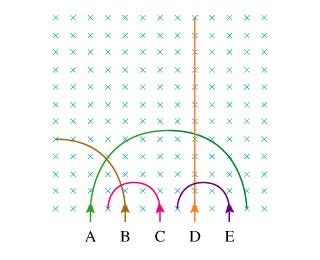# Ranking velocities of particles in uniform magnetic field

• Linus Pauling

#### Linus Pauling

1. Five equal-mass particles (A–E) enter a region of uniform magnetic field directed into the page. They follow the trajectories illustrated in the figure.Rank from largest to smallest velocity

2. qvBsin(theta) = ma
qvB = ma = m(v^2/r)
v = qBr/m

3. Based on their radii, it should be A > B > C=E

But what about D? It is neutral, so has no radius because it doesn't go in a circle, but clearly has a velocity despite r = 0. Is the answer indeterminate, then?

I think you're meant to assume that all particles have the same charge; otherwise, the problem would be unsolvable. So assuming they have the same charge, D appears to go straight because it's barely affected by the magnetic field. What does that tell you about its velocity?

The answer actually was that it couldn't be determined.

Now I am asked to rank the speeds largest to smallest of A, B, C, and E. I thought it was clearly A > B > C=E, but it is not and it tells me this:

The radius of the trajectory of a particle depends on more than just its speed. Be sure not to make assumptions about the relative magnitudes of the charges.

WTF?

v = qBr/m
The velocity depends on the charge, and you are not given any information about the charges. Therefore the problem is not solvable.

nvmm

Last edited: